### Home > MC1 > Chapter 3 > Lesson 3.4.5 > Problem3-153

3-153.
1. . Express each of the following number as a product of its prime factors. Use exponents to represent repeated multiplication, when applicable. An example is given below. Homework Help ✎ 40 = 2 · 20 = 2 · 2 · 10 = 2 · 2 · 2 · 5 = 23 · 5

1. 30

2. 300

3. 17

4. 21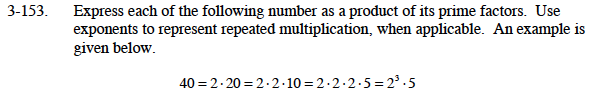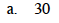Use the example above as a guide if you are having trouble. You can also refer to problem 3-64 for help with prime factorization.

2 · 3 · 5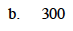This problem is very similar to part (a). It may also be helpful to think about how 300 relates to 30. What prime factors could you multiply by 30 to get 300?

22 · 3 · 52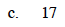Is there any way to break 17 down to prime factors? It is possible for a number to be the only prime factor of itself.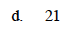Follow the steps you did for parts (a) and (b).